Class 10 Mathematics: CBSE Sample Question Paper- Term I (2021-22) - 1

# Class 10 Mathematics: CBSE Sample Question Paper- Term I (2021-22) - 1 Notes | Study CBSE Sample Papers For Class 10 - Class 10

## Document Description: Class 10 Mathematics: CBSE Sample Question Paper- Term I (2021-22) - 1 for Class 10 2022 is part of CBSE Sample Question Papers for 2021-22 for CBSE Sample Papers For Class 10 preparation. The notes and questions for Class 10 Mathematics: CBSE Sample Question Paper- Term I (2021-22) - 1 have been prepared according to the Class 10 exam syllabus. Information about Class 10 Mathematics: CBSE Sample Question Paper- Term I (2021-22) - 1 covers topics like Class-XTime: 90 MinutesM.M: 40, Section - A, Section - B, Section - C and Class 10 Mathematics: CBSE Sample Question Paper- Term I (2021-22) - 1 Example, for Class 10 2022 Exam. Find important definitions, questions, notes, meanings, examples, exercises and tests below for Class 10 Mathematics: CBSE Sample Question Paper- Term I (2021-22) - 1.

Introduction of Class 10 Mathematics: CBSE Sample Question Paper- Term I (2021-22) - 1 in English is available as part of our CBSE Sample Papers For Class 10 for Class 10 & Class 10 Mathematics: CBSE Sample Question Paper- Term I (2021-22) - 1 in Hindi for CBSE Sample Papers For Class 10 course. Download more important topics related with CBSE Sample Question Papers for 2021-22, notes, lectures and mock test series for Class 10 Exam by signing up for free. Class 10: Class 10 Mathematics: CBSE Sample Question Paper- Term I (2021-22) - 1 Notes | Study CBSE Sample Papers For Class 10 - Class 10
 1 Crore+ students have signed up on EduRev. Have you?

Class-X
Time: 90 Minutes
M.M: 40

General Instructions:

1. The question paper contains three parts A, B and C.
2. Section A consists of 20 questions of 1 mark each. Any 16 questions are to be attempted
3. Section B consists of 20 questions of 1 mark each. Any 16 questions are to be attempted
4. Section C consists of 10 questions based on two Case Studies. Attempt any 8 questions.
5. There is no negative marking.
Section - A

Q.1: The smallest number which when divided by 26 and 32 leaves remainders 6 and 12 respectively is:
(a) 416
(b) 420
(c) 396
(d) 436

∵ 26 – 6 = 20 and 32 – 12 = 20
Now,  26 = 2 × 13 and 32 = 2 × 2 × 2 × 2 × 2

∴ LCM (26, 32) = 25 × 13 = 416

Required number = LCM (26, 32) – 20 = 416 – 20

= 396.

Q.2: If in two triangles DEF and PQR, ∠D = ∠Q and∠R = ∠E, then which of the following is not true?

(a)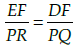(b)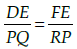(c)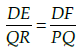(d)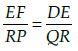In ΔDEF and ΔPQR, ∠D = ∠Q and ∠R = ∠E. By AA similarity,
we get ΔDEF ~ ΔQRP.
Hence,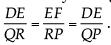Q.3: If the points P(2, 4) is equidistant from the points A(5, k) and B(k, 7), then the value of k is:
(a) 3
(b) 2
(c) 1
(d) – 3

Distance of AP = Distance of BP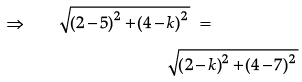⇒ (– 3)2 + (4 – k)2 = (2 – k)2 + (– 3)2
⇒ 16 + k2 – 8k = 4 + k2 – 4k
⇒ –4k = –12
⇒ k = 3.

Q.4: If 3x + y = 14 and 2x – y = 1, then the value of y is:
(a) 2
(b) 3
(c) 4
(d) 5

3x + y = 14   ...(i)
and 2x – y = 1   ...(i)
⇒ x = 3
Putting x = 3 in eq. (i), we get
9 + y = 14
⇒ y = 14 – 9 = 5.

Q.5: If the distance between the points (x, b) and (a, 0) is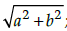then the value of x is: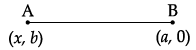(a) a
(b) b
(c) 2a
(d) 2b

Distance between the points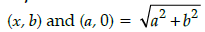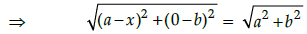⇒ (a – x)2 + b = a2 + b2

⇒ a2 + x2 – 2ax = a2

⇒ x2 – 2ax = 0

⇒ x(x – 2a) = 0

⇒ x = 0, 2a.

Q.6: In the equation 2x + 3y = 13, if x is having a value 2, then corresponding value of y is
(a) 2
(b) 3
(c) 1
(d) 4

Putting x = 2 in equation
2x + 3y = 13, we get
2 × 2 + 3y = 13
⇒ 3y = 13 – 4 = 9
⇒ y = 3.

Q.7: If (4, 10) is the mid point of the line segment joining (– 8, 13) and (x, 7), then x is equal to:
(a) 16
(b) 10
(c) 8
(d) 4

Here, x1 = – 8, y1 = 13

and x2 = x, y2 = 7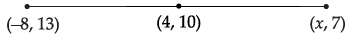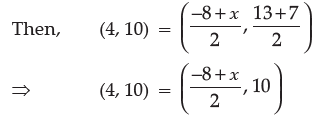Comparing both sides, we get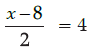⇒ x – 8 = 8

i.e., x = 16.

Q.8: If the mid point of the line segment joining the points A(5, 4) and B(k, 8) is P(x, y) and 2x + 3y – 10 = 0, then the value of k is:
(a) 12
(b) – 12
(c) 13
(d) – 13

Mid point of A (5, 4) and B(k, 8)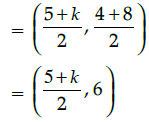which is equal to P(x, y)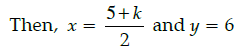∵  2x + 3y – 10 = 0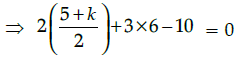⇒ 5 + k + 8 = 0

⇒ k = – 13.

Q.9: Aman and Nitin are two friends. One day, they discuss about different concepts of circles and squares. And they try to solve the problem which is given below :
If the perimeter of a circle is equal to that of a square, then the ratio of their areas is:
(a) 22 : 7
(b) 14 : 11
(c) 7 : 22
(d) 11 : 14

Let the radius of circle be ‘r’ and side of square be ‘a’.
According to given question,
Perimeter of circle = Perimeter of square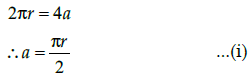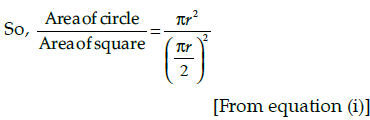Solving equation (i), we get result as 14/11.

Q.10:  If tan θ + cot θ = 7, then the value of tan2 θ + cot2 θ is:

(a) 48
(b) 49
(c) 47
(d) 46

Given,

tan θ + cot θ = 7

Taking square on both sides, we get

tan2θ + cot2θ + 2 tan θ . cot θ = 49

⇒ tan2θ + cot2θ + 2 = 49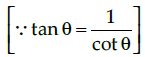⇒ tan2 θ + cot2 θ = 49 – 2 = 47.

Q.11: The area of circle that can be inscribed in a square of side 1.4 cm, is:
(a) 2.2 cm
(b) 1.82 cm
(c) 1.62 cm
(d) 1.54 cm2

side of a square = diameter of the circle

∴ Radius of the circle (r) = 1.4/2

= 0.7 cm

Now, area of the circle = πr2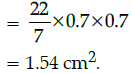Q.12: In the figure given below, two line segments AC and BD intersect each other at the point P such that PA = 6 cm, PB = 3 cm, PC = 2.5 cm, PD = 5 cm, ∠APB = 50° and ∠CDP = 30°. Then, ∠PBA is equal to: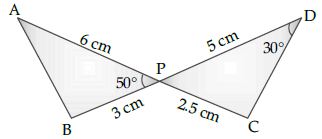(a) 50°
(b) 30°
(c) 60°
(d) 100°

In the given figure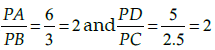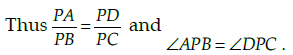By SAS similarity, we get ΔAPB ~ ΔDPC.

Hence, ∠PBA = 100°.

Q.13: The value of sin2 45° cos2 30° + 1/2 cos2 90° – 2 cot2 30° + 4 sec2 60°.
(a) 83/8
(b) 83/7
(c) 81/8
(d) 83/5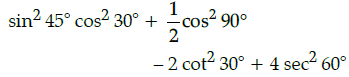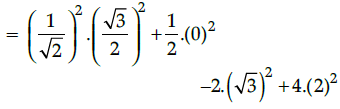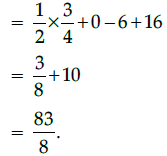Q.14: A box contains cards numbered 1 to 70. A card is drawn at random from the box. The probability that the drawn card has a number which is a perfect cube is:
(a) 1/7
(b) 2/35
(c) 1/14
(d) 3/70

Given numbers = 1, 2, 3, 4, 5, ...., 70
i.e., total outcomes,
n(S) = 70
Perfect cube numbers between 1 to 70 = 8, 27, 64
i.e., favourable outcomes,
n(E) = 3

∴ Required probability,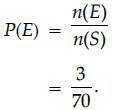Q.15: The value of k for which the system of equations 4x + y = 5 and 8x + ky = 12 has not solution, is:
(a) 10
(b) 5
(c) 2
(d) 1

System has no solution if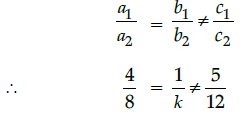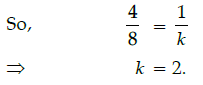Q.16: If cos A =4/5 then the value of tan A is:
(a) 3/5
(b) 3/4
(c) 4/3
(d) 1/8

Given, cos A =4/5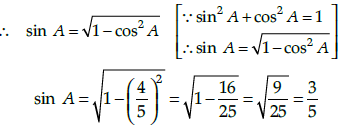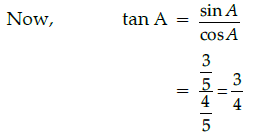Q.17: If 2 sin 4θ = 1/2, then the value of θ is :
(a) 15º
(b) 36º
(c) 45º
(d) 60º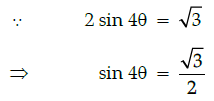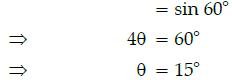Q.18: Rahul is a class X student, he love to play with marbles. He has a bag containing 10 blue, 7 white and 8 red marbles. He thought that, he will win game only when he draw white marble. Then what will be the probability that he will win the game.
(a) 17/25
(b) 18/25
(c) 3/5
(d) 11/25

Total number of marbles

= 10 + 7 + 8
= 25
i.e.,   n(S) = 25
Number of non-white marbles
= 10 + 8 + 18
i.e., n(E) = 18
P(getting a non white marble)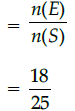Q.19: If the length of the minute hand of a clock is 10 cm, then the area swept by the minute hand from 9.10 a.m. to 9.45 a.m., is:
(a) 183.17 cm2
(b) 184.17 cm2
(c) 182.17 cm2
(d) 185.17 cm2

Since, angle subtended in 1 minute = 6°
So, angle subtended in 35 minutes
= 35 × 6°
= 210°
Now, area swept by the minute hand = area of the sector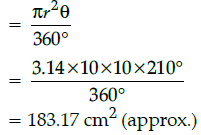Q.20: The area of minor segment of a circle of radius 7 cm, when its central angle is 30, is:
(a) 5/12 cm2
(b) 1/2 cm2
(c) 7/12 cm2
(d) 11/12 cm2

Given, r = 7 cm and q = 30°

Then, the area of minor segment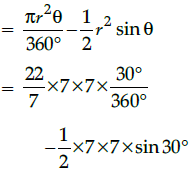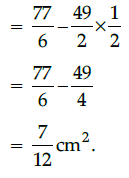Section - B

Q.21: In the given figure, if ABCD is a rectangle, then area of the circle is :

[Using, π = 3.14]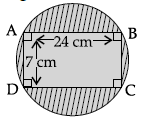(a) 492 cm2
(b) 492.625 cm2
(c) 432.625 cm2
(d) 490.625 cm
2

Here, AB = 24 cm and AD = 7 cm.

and BD is a diagonal of rectangle ABCD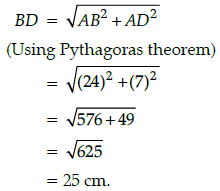∵ Diameter of the circle = Diagonal BD of ABCD

i.e., 2r = 25

⇒ r  = 25/2 cm
Area of the circle = πr2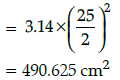Q.22: If 2x – y = 4, y – z = 6 and x – z = 10, then x is :
(a) – 4
(b) 3
(c) 0
(d) 4

2x – y = 4 ...(i)

y – z = 6 ...(ii)

and x – z = 10 ...(iii)

From eq. (ii), we get

z = y – 6

Putting the value of z in eq. (iii), we get

x – y = 4 ...(iv)

Solving eq. (i) and (iv), we get

x = 0 and y = – 4

Q.23: In DABC, if DE || BC, then the value of x is :
(a) 1
(b) 2
(c) 3
(d) 4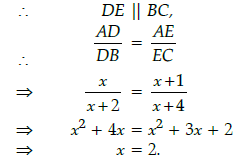Q.24: If two zeroes of the polynomial p(x) = x3 – 4x2 – 3x + 12 are 2 and −√2, then its third zero is:
(a) 4
(b) 5
(c) 6
(d) 1

Let α, β and γ are zeroes

of given polynomial, then α = √2 and β = − √2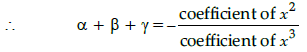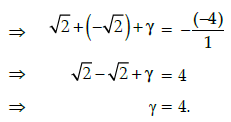Q.25: The square of radius of a circle whose area is equal to the difference of the areas of the two circlces of radii 6 cm and 4 cm is:
(a) 16π cm
(b) 20 cm

(c) 8π cm
(d) 2π cm

Let r1 = 6 cm

and r2 = 4 cm.

According to question,

Area of circle = area of first circle – area of second circle.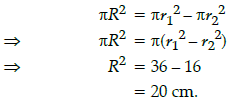Q.26: It is given that DABC ~ DPQR with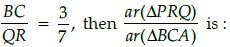(a) 49/9
(b) 49/4
(c) 47/4
(d) 47/9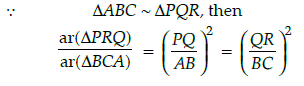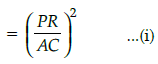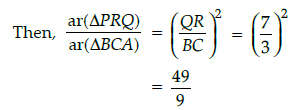Q.27: If two zeroes of the polynomial p(x) = x3 – 5x2 – 16x + 80 are equal in magnitude but opposite in sign, then third zero is :
(a) 5
(b) 3
(c) –2
(d) 4

Let α, β and γ be the zeroes of given polynomial

p(x) = x3 – 5x2 – 16x + 80, such that α+ β = 0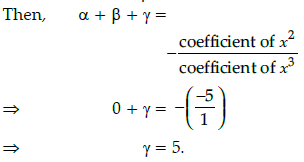Q.28: If sin θ =3/5, then the value of sec q is:
(a) 4/5
(b) 3/4
(c) 1/5
(d) 5/4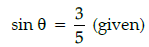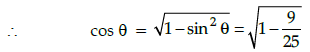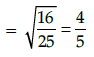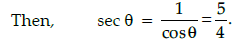Q.29: If E be an event such that P(E) = 10/11 , then P(not E) =
(a) 10/11
(b) 11/10
(c) 1/11
(d) 1/10

Given P(E) = 10/11
So, P(not E) = 1 – P(E)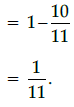Q.30: If 5 cosec θ = 7, then the value of sin3 θ + sin2 θ + sin θ is:
(a) 109/343
(b) 545/343
(c) 107/343
(d) 113/343

∵ 5 cosec θ = 7 (given)

⇒ cosec θ =7/5
or sin θ = 5/7
Now, sin3 θ + sin2 θ + sin θ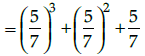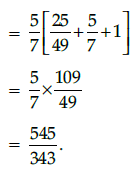Q.31: The HCF of 1500, 2500 and 3000 is:
(a) 600
(b) 500
(c) 800
(d) 400

In case of assertion:

1500 = 22 × 3 × 53

2500 = 22 × 54

3000 = 23 × 3 × 53

HCF (1500, 2500 and 3000)

= 22 × 53

= 500

Q.32: If radius of a circle is 3 cm and of angle 60° at the centre, then the length of the arc is:
(a) 3.14 cm
(b) 6.28 cm
(c) 31.4 cm
(d) 62.8 cm

Here, r = 3 cm and q = 60°.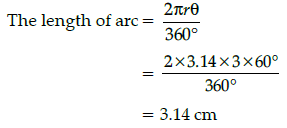Q.33: The greatest number of four digits exactly divisible by 15, 20 and 30 is :
(a) 9950
(b) 9940
(c) 9960
(d) 9970

LCM of 15, 20 and 30 is 60 and the largest 4

digit number is 9999,

which divides by 60, get remainder 39.

So, the required number = 9999 – 39 = 9960.

Q.34: In a lottery, there are 8 prizes and 16 blanks, then the probability of not getting a prize is:
(a) 1/3
(b) 2/3
(c) 1
(d) 0

Total Possible outcomes = 8 + 16 = 24.

No. of prizes = 8

∴ Probability (getting not a prize)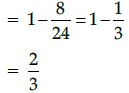Q.35: If P(5, 3), Q(11, 5) and R(2, 7) be the vertices of DPQR and PT is a median, then the coordinates of T are:
(a)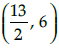(b)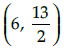(c) (3,4)
(d)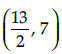Since PT is a median, then T is the mid point of QR.

∴ Coordinates of T are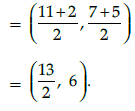Q.36: If the zeroes of the polynomial p(x) = x3 – 5x2 + 2x + 3 are x, x + y and x – y,

then the value of x is:
(a) 1/3
(b) 2/3
(c) 5/3
(d) 4/3

Since x, x + y and x – y are zeroes of p(x).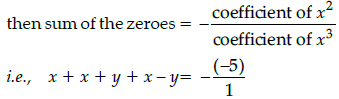∴ 3x = 5
⇒ x= 5/3

Q.37: If AB =9 cm, BC = 6 cm then AB2 + BC2 is:
(a) 115
(b) 118
(c) 117
(d) 121

Given, AB = 9 cm

and BC = 6 cm

Then, AB2 + BC2 = (9)2 + (6)2

= 81 + 36 = 117

Q.38: 3 group of children decided to calculate the length of park model designed by them for school project. The given below figure is as shown then what is the value of x ?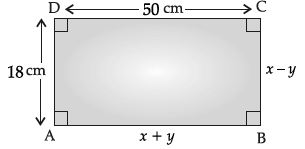(a) 35 cm
(b) 36 cm
(c) 37 cm
(d) 34 cm

Since ABCD is a rectangle,
AB = CD
i.e., x + y = 50 ...(i)
i.e., x – y = 18 ...(ii)
On adding eq. (i) and (ii), we get
2x = 68
⇒ x = 34 cm.

Q.39: If √3 is an irrational number, then 5√3 is a/an:
(a) rational number
(b) prime number

(c) odd number
(d) irrational number

5√3 is not a rational number because the product of rational and irrational number is irrational

Q.40: If sin θ + cos θ = √3, then the value of sin θ cos θ is:
(a) 1
(b) - 1
(c) 2
(d) 3

Given sin θ + cos θ = √3

On squaring both the sides, we get
sin2 θ + cos2 θ + 2 sin θ cos θ = 3
⇒ 1 + 2 sin θ cos θ = 3
⇒ 2 sin θ cos θ = 2
⇒ sin θ cos θ = 1.

Section - C

Case Study - 1

The figure given alongside shows the path of a diver, when she takes a jump from the diving board. Clearly it is a parabola.
Annie was standing on a diving board, 48 feet above the water level. She took a dive into the pool. Her height (in feet) above the water level at any time ‘t’ in seconds is given by the polynomial h(t) such that h(t) = –16t² + 8t + k.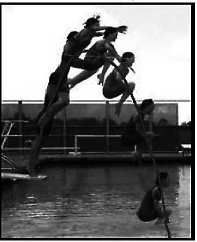Q.41: Rita’s height (in feet) above the water level is given by another polynomial p(t) with zeroes –1 and 2. Then p(t) is given by-

(a) t² + t – 2
(b) t² + 2t – 1
(c) 24t² – 24t + 48
(d) – 24t² + 24t + 48

t= –1 & t=2 are the two zeroes of the polynomial p(t)

Then p(t)=k (t– (–1))(t–2) = k(t +1)(t–2)

When t = 0 (initially) h1 = 48ft

p(0)=k(02– 0 –2)= 48

i.e. –2k = 48

So the polynomial is -24(t²- t -2) = -24t² + 24t + 48.

Q.42: The zeroes of the polynomial r(t) = – 12t² + (k – 3)t + 48 are negative of each other. Then k is
(a) 3
(b) 0
(c) – 1.5
(d) – 3

When the zeros are negative of each other,
sum of the zeros = 0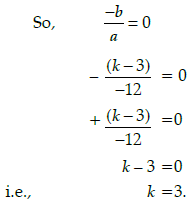Q.43: What is the value of k?
(a) 0
(b) – 48
(c) 48
(d) 48/–16

Initially, at t=0, Annie’s height is 48ft

So, at t =0, h should be equal to 48

h(0) = –16(0)² + 8(0) + k = 48

So k = 48

Q.44: A polynomial q(t) with sum of zeroes as 1 and the product as –6 is modelling Anu’s height in feet above the water at any time t( in seconds). Then q(t) is given by
(a) t² + t + 6
(b) t² + t – 6

(c) – 8t² + 8t + 48
(d) 8t² – 8t + 48

A polynomial q(t) with sum of zeroes as 1

and the product as –6 is given by q(t) = k(t² - (sum of zeroes)t + product of zeroes)

= k(t² –1t + (–6)) ………..(1)
When t=0 (initially) q(0)= 48ft
q(0) = k(02–1 (0)–6)=48

i.e. –6k = 48 or k =–8

putting k=–8 in equation (1),

required polynomial is.

=–8 (t2–1t–6)

=–8 t2 + 8t +48

Q.45: At what time will she touch the water in the pool?
(a) 30 seconds
(b) 2 seconds
(c) 1.5 seconds
(d) 0.5 seconds

When Annie touches the pool, her height =0 feet

i.e. –16t² + 8t + 48 =0 above water level
2t² – t –6 = 0
2t² - 4t +3t -6 = 0
2t(t-2) +3(t–2) = 0
(2t +3) (t–2) = 0

i.e. t = 2 or t= –3/2

Since time cannot be negative , so t = 2 seconds

Case Study - 2

A hockey field is the playing surface for the game of hockey. Historically, the game was played on natural turf (grass) but nowadays it is predominantly played on an artificial turf. It is rectangular in shape - 100 yards by 60 yards. Goals consist of two upright posts placed equidistant from the centre of the backline, joined at the top by a horizontal crossbar. The inner edges of the posts must be 3.66 metres (4 yards) apart, and the lower edge of the crossbar must be 2.14 metres (7 feet) above the ground. Each team plays with 11 players on the field during the game including the goalie. Positions you might play include-
Forward: As shown by players A, B, C and D.

• Midfielders: As shown by players E, F and G.
• Fullbacks: As shown by players H, I and J.
• Goalie: As shown by player K

Using the picture of a hockey field below, answer the questions that follow: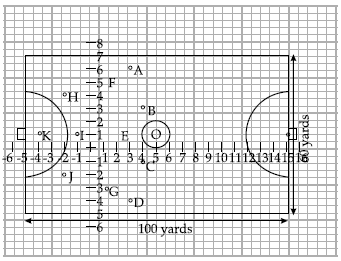Q.46: The point on x axis equidistant from I and E is 1
(a) (1/2, 0)
(b) (0, – 1/2)

(c) (– 1/2, 0)
(d) ( 0, 1/2)

Let the point of × axis equidistant from I (–1, 1) and E(2,1) be (x, 0)
Then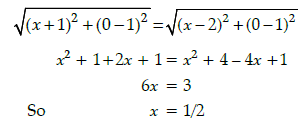∴ The required point is (1/2, 0)

Q.47: The coordinates of the centroid of ΔEHJ are
(a) (– 2/3, 1)
(b) (1, – 2/3)
(c) (2/3, 1)
(d) ( – 2/3, – 1)

Centroid of ΔEHJ with E(2, 1), H(–2, 4) & J(–2, –2) is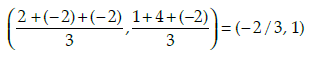Q.48: The point on y axis equidistant from B and C is
(a) (– 1, 0)
(b) (0, – 1)

(c) (1, 0)
(d) ( 0, 1)

Let the point of y axis equidistant from B(4, 3) and C(4, – 1) be (0, y)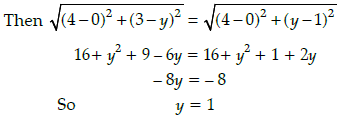∴The required point is (0, 1)

Q.49: If a player P needs to be at equal distances from A and G, such that A, P and G are in straight line, then position of P will be given by
(a) (– 3/2, 2)
(b) (2, – 3/2)
(c) (2, 3/2)
(d) ( – 2, – 3)

If P needs to be equal distance from A(3, 6) and G(1, –3), such that A, P and G are collinear, than P will be the mid-point of AG.
So coordinates of P will be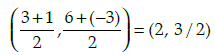Q.50: What are the coordinates of the position of a player Q such that his distance from K is twice his distance from E and K, Q and E are collinear?
(a) (1, 0)
(b) (0, 1)
(c) (– 2, 1)
(d) (– 1, 0)

Let the coordinates of the position of a player Q such that his distance from K(–4, 1) is twice his distance from E(2, 1) be Q(x, y)
Then KQ : QE = 2 : 1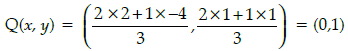The document Class 10 Mathematics: CBSE Sample Question Paper- Term I (2021-22) - 1 Notes | Study CBSE Sample Papers For Class 10 - Class 10 is a part of the Class 10 Course CBSE Sample Papers For Class 10.
All you need of Class 10 at this link: Class 10

## CBSE Sample Papers For Class 10

174 docs|7 tests
 Use Code STAYHOME200 and get INR 200 additional OFF

## CBSE Sample Papers For Class 10

174 docs|7 tests

Track your progress, build streaks, highlight & save important lessons and more!

,

,

,

,

,

,

,

,

,

,

,

,

,

,

,

,

,

,

,

,

,

;